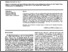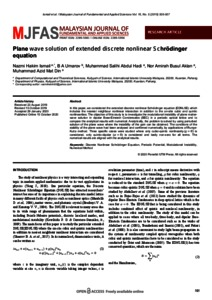# Plane wave solution of extended discrete nonlinear Schrödinger equation

Ismail, Nazmi Hakim and Umarov, Bakhram and Abdul Hadi, Muhammad Salihi and Mohd Busul Aklan, Nor Amirah and Mat Din, Muhammad Azid (2020) Plane wave solution of extended discrete nonlinear Schrödinger equation. Malaysian Journal of Fundamental and Applied Sciences, 16 (5). pp. 505-507. ISSN 2289-5981 E-ISSN 2289-599XPreview
PDF - Published Version

## Abstract

In this paper, we considered the extended discrete nonlinear Schrödinger equation (EDNLSE) which includes the nearest neighbour nonlinear interaction in addition to the on-site cubic and quintic nonlinearities. The objective of this study is to investigate the modulational instability of plane matterwave solution in dipolar Bose-Einstein Condensates (BEC) in a periodic optical lattice and to compare the analytical results with numerical. Analytically, the problem is solved by using perturbed solution of the plane wave where the instability of the gain can be obtained. The conditions of the stability of the plane wave had been analysed and confirmed numerically, by applications of Runge- Kutta method. Three specific cases were studied where only cubic-quintic nonlinearity(q = 0) is considered, only quintic-dipolar (alpha = 0) is considered and lastly non-zero for all terms. The numerical results are aligned with the analytical results.

Item Type: Article (Journal) 3938/86865 Discrete Nonlinear Schrödinger Equation, Periodic Potential, Modulational Instability, Numerical Method Q Science > QA MathematicsQ Science > QC Physics Kulliyyah of Science > Department of PhysicsKulliyyah of Science > Department of Computational and Theoretical Sciences Dr Bakhram Umarov 28 Dec 2020 15:32 28 Dec 2020 15:32 http://irep.iium.edu.my/id/eprint/86865View Item